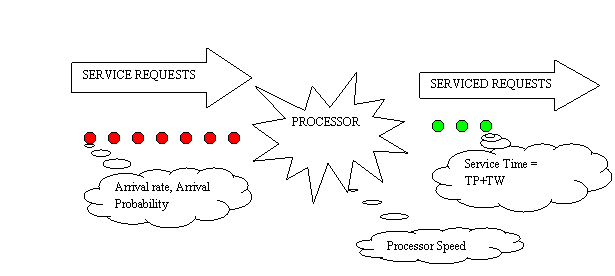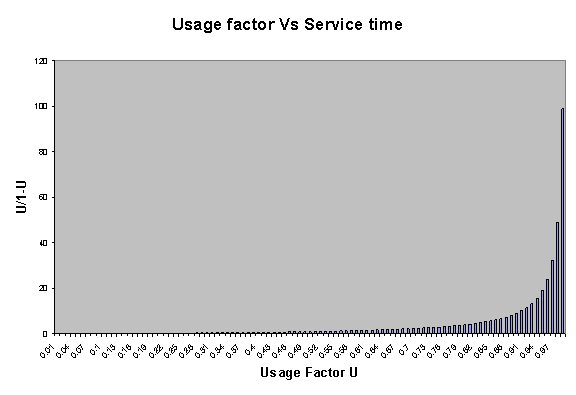USAGE FACTOR, QUEUING THEORY BASICS AS IT APPLIES TO SYSTEM PERFORMANCE.

In this write up I have tried to give a very basic overview of queuing theory concepts, as it applies to system performance.

Queuing Theory is a set of mathematical solutions to waiting line or process type problems. In these problems, there is some process which consumes time, units arrive in the system, and are processed though it.A simple queue can be thought of as a set of requests that need servicing arriving at a particular rate with a particular probability of arrival (red dots). A processor that services the request at a particular speed and serviced requests (green dots).

The arrival rate and the probability determine the input queue length at any given time. For further reading you can try a google search on queuing theory.

What we are interested in, is the Total elapsed time which is the sum of  queue wait time and the request processing time.  For the sake of simplicity let us assume that  all the arriving requests are of equal complexity, which means that each request takes the same time to process when it is the only request to be processed.

In most real life situations the processor is shared among requesters. ( Only a few tellers for a queue of customers at the bank, Only one CPU complex for thousands of SQL request, Only One VSAM dataset on a disk for thousands of SQL on that table).

The questions most of  us would like to answer are ,

If one SQL when run by itself takes "m" milliseconds to run , how long will 5000 requests take to run?
Is it 5000 x "m" milliseconds? If so how long will 10000 SQLs take? Definitely it is not 10000 x "m" milliseconds. Or is it? Is there a point at which your processor becomes overwhelmed  and simply breaks down?  If  so, where is that point? How many SQLs can I run concurrently with out reaching the point of melt down? etc.

To understand and answer business critical questions like these, one needs to have at least a basic understanding of  resource usage factor, how it affects service times etc.

Usage factor U , very simply put is the ratio of the current usage of a resource to it's maximum available usage.

A 100 GB disk which has 60 GB data in it has a usage factor of  0.6 .
A 1000 MIPS CPU complex  which has applications running on it that consume 750 MIPS has a usage factor of 0.75.
A  Truck that can carry 5000 Kg with a maximum axle rating of 10000 Kg has a usage factor of 0.5.
In our queue example, if the processor has a capacity to service 1000 requests per second and if it has 1000 requests every second  to process it has a usage factor  of  1.0

Understanding the effect on usage factor is key in estimating the point of melt down.

Total  Elapsed time = Total  Queue Wait time + Actual Request Service time

Request Service time =  ( Ideal Request Service time x  Usage factor ) / (1 - Usage factor )    where   0>=  Usage factor >= 1

The  Request Service time is proportional to U/(1-U).

When we invest in infrastructure we want to get the maximum return on our investment. Naturally, we are tempted to use the resource close to it's maximum rated capacity. Or a usage factor of  close to 1.

Let us look at what happens to service time as we approach a usage factor of 1 . U/(1-U)   approaches 1/(1-1) which is 1/0 .

Therefore as U  ==> 1 ,  U/1-U  ==> Infinity . Hence your service time also approaches infinity.As you can see from the simple plot above as U reaches 0.95  you are fast approaching the meltdown point.

As U gets closer to .95  the Service time of the system reacts violently and starts approaching infinity.

The "system"  might be your  CPU , DISK, Network, employee or your motor car.

It is just a bad idea to push the average resource utilization factor beyond 0.9, and the peak resource utilization factor beyond 0.95.

The next time some one in your company tells you to use a DASD volume to it's full capacity or gives you just enough buffers for your requirement think about the meltdown point where U approaches unity.